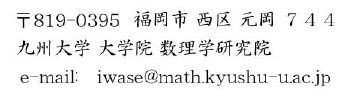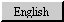## \$B4d@%(B \$BB'IW(B \$B!J\$\$\$o\$;(B \$B\$N\$j\$*!K!w(B \$B6eBg(B \$B?tM}(B \$B!J?t3XItLg!K(B\$B%&%'%V%Z!<%8!'(B

• \$B?t3X\$H4X78\$J\$\$8D?M\$N#H#P\$G\$9!J30It%j%s%/!K(B
• \$B8&5f=82q!V(BBuilding-Up Differentiable Homotopy Theory 2020 in Shinshu\$B!W!J>>K\!\$#2#0G/#37n!K(B
• \$B8&5f=82q!V(BBuilding-Up Differentiable Homotopy Theory\$B!W!JJ!2,!\$#1#9G/#37n!K(B
• \$B8&5f=82q!VHs0BDj%[%b%H%T!
• \$BBh#3#72sJQ4972O@%7%s%]%8%&%`!JJ!2,!\$#1#0G/#1#17n!K(B
• \$B%[%b%H%T!
• \$BBh(B4\$B2s(B \$BJ!2,;%KZ(B \$B4v2?3X%;%_%J!<(B (\$BJ!2,!\$#1#0G/#27n(B)
• \$BBh(B3\$B2s(B Lusternik-Schnirelmann \$B%+%F%4%j!<%7%s%]%8%&%`!JEbDE!\$#0#8G/#1#07n!K(B
• \$B8&5f=82q!V%[%b%H%T!.8&5f2q!W!JJ!2,!\$#0#8G/#37n!K(B
• \$BBh(B54\$B2s%H%]%m%8!<%7%s%]%8%&%`!J2qDE!\$#0#7G/#87n!K(B
• \$BBh(B2\$B2s(B Lusternik-Schnirelmann \$B%+%F%4%j!<%7%s%]%8%&%`!JEbDE!\$#0#6G/#1#0!<#1#17n!K(B
• \$B8&5f=82q!V<+8J%[%b%H%T!<=89g\$H\$=\$N<~JU!W!J@iMU!\$#0#2G/#1#27n!K(B
• \$B2CF#==5H@h@84TNq5-G08&5f=82q!JJ!2,!\$#0#2G/#1#17n!K(B

• \$BJXMxH"!'(B
• \$B\$I\$3\$G\$b(B XeTeX \$B;O\$a\$^\$7\$?(B\$B!J1|B<\$5\$s\$N%9%/%j%W%H\$K\$h\$k!K(B
• \$B\$I\$3\$G\$b(B TeX \$B;O\$a\$^\$7\$?(B\$B!J1|B<\$5\$s\$N%9%/%j%W%H\$K\$h\$k!K(B
• \$B;vL3
• \$B:G6a;#\$C\$?

• \$BF|K\?t3X2q9V1iMQ%"%V%9%H%i%/%H(Bplatex\$BMM<0%U%!%\$%k(B
• \$B%"!<%+%\$%V!J(BXeLaTeX \$B4XO"\$N%U%!%\$%k\$J\$I!K(B
• PukiWiki\$B!V\$\$\$H\$K\$-!W(B
• \$B<+Bp%5!<%P(B

• \$B8D?M>pJs!'(B
• PukiWiki\$B!V\$\$\$H\$K\$-(B/\$B3hF0M=Dj!W(B
• \$B?tM}3X8&5f1!%9%?%C%U>R2pJG(B
• \$B8&5fpJs!J650i8&5f3hF0!K(B

• \$BO@J8%j%9%H(B
• \$B3hF05-O?(B
• PGP\$B8x3+80(B (Norio.iWASE.E2E82BDB)

• 2016\$BG/EYA04|(B \$B@~7?Be?t3XB3O@9V5AMQ%N!<%H(B
• 2016\$BG/EYA04|(B \$B1~MQJ#AG4X?tO@9V5AMQ%N!<%H(B
• \$B#0#8G/EYA04|(B \$B=8Cf9V5A(B Lusternik-Schnirelmann \$B%+%F%4%j?t\$H0LAjE*J#;(\$5(B \$B1wEl5~9)6HBg3X!J(BXeLaTeX \$B;H\$C\$F\$^\$9(B, Last updated: 15th/Jun/2016\$B!K(B
• \$B#0#7G/#37n!V(BENCOUNTER with MATHEMATICS \$BBh(B39\$B2s(B Lusternik-Schnirelmann \$B%+%F%4%j!\$B!!(B\$B!c(BLusternik-Schnirelmann \$B%+%F%4%j?t!d!u!c(BLusternik-Schnirelmann \$B%+%F%4%j?t\$H(B A-\$B9=B\$!d(B\$B!J(BXeLaTeX \$B;H\$C\$F\$^\$9(B, updated 7th/Mar/2007\$B!K(B
• \$B#0#6G/EY8e4|(B \$B=8Cf9V5A(B A \$B9=B\$\$H(B L-S \$B\$NG-(B \$B1wKL3\$F;Bg3X!J(BXeLaTeX \$B;H\$C\$F\$^\$9(B, Last updated: 5th/Mar/2007\$B!K(B
• \$B#0#6G/#77n(B \$B?tM}8x3+9V:B(B \$B!cG4EZ\$O\$\$\$/\$D!)!d(B\$B!J(BXeLaTeX \$B;H\$C\$F\$^\$9(B, updated 26th/Jul/2006\$B!K(B
• \$B#0#6G/EY(B \$BBg3X1!9V5A(B A \$B9=B\$\$H(B L-S \$B\$NG-\$NOC!&%Q!<%H#1(B\$B!J(BXeLaTeX \$B;H\$C\$F\$^\$9(B, updated 7th/Jun/2006\$B!K(B
• \$B#0#6G/EY(B \$BBg3X1!9V5A(B A \$B9=B\$\$H(B L-S \$B\$NG-\$NOC!&%Q!<%H#2(B\$B!J(BXeLaTeX \$B;H\$C\$F\$^\$9(B, updated 7th/Jun/2006\$B!K(B
• \$B#0#6G/EY(B \$BBg3X1!9V5A(B A \$B9=B\$\$H(B L-S \$B\$NG-\$NOC!&J88%I=(B\$B!J(BXeLaTeX \$B;H\$C\$F\$^\$9(B, updated 7th/Jun/2006\$B!K(B
• \$B#0#4G/EY8e4|(B \$B=8Cf9V5A(B A \$B9=B\$\$H(B L-S \$B\$NG-(B \$B1w2,;3Bg3X!J(BLast updated: 9th/Jan/2005\$B!K(B
• \$B#0#2G/EY8e4|(B \$B=8Cf9V5A(B A \$B9=B\$\$H(B L-S \$B\$NG-(B \$B1w5~ETBg3X!J(BLast updated: 16th/Mar/2003\$B!K(B

• \$B9V1i:Q#O#H#P%7!<%H!J(BAdobe PDF / QuickTime Movie \$B%U%!%\$%k!K!'(B
• \$B#1#0G/(B \$B@\?(9=B\$!"FC0[E@!"HyJ,J}Dx<05Z\$S\$=\$N<~JU!JF6Ll8PJ82=%;%s%?! pdf)
• \$B#0#8G/(B Groups of Self-Homotopy Equivalences and Related Topics (Halifax, Canada) \$B\$G\$N9V1i(B (beamer + xelatex => pdf)
• \$B#0#7G/(B Conference on "Algebraic Topology: old and new - M.M.Postnikov Memorial Conference" (Bedlewo, Poland)\$B\$G\$N9V1i(B (beamer + latex => pdf)
• \$B#0#6G/(B International Conference on Algebraic Topology (Henan University, Kaifeng, China)\$B\$G\$N9V1i(B (beamer + platex => pdf)
• \$B#0#5G/(B Conference on "Stable and algebraic homotopy" (Schloss Ringberg, Germany)\$B\$G\$N9V1i(B (pdf on powerpoint => qt)
• \$B#0#4G/(B III Joint meeting Japan-Mexico in Topology and its applications (Oaxaca, Mexico)\$B\$G\$N9V1i(B (latex w/bigfont => pdf)
• \$B#0#4G/(B International Conference on Algebraic Topology (Chinese Academy of Science, China)\$B\$G\$N9V1i(B (latex w/bigfont => pdf)
• \$B#0#3G/(B Lusternik-Schnirelmann Category and Related Topics (\$BErI[1!%O%\$%D(B)\$B\$G\$N9V1i(B (platex w/bigfont => pdf)
• \$B#0#3G/(B General and Geometric Topology and Related Topics (\$B5~ETBg3X?tM}2r@O8&5f=j(B)\$B\$G\$N9V1i(B (platex w/bigfont => pdf)
• \$B#0#2G/(B Topology in Matsue, International Conference on Topology and its Applications Joined with Japan-Mexico Topology Symposium (\$BEg:,Bg3X(B) \$B\$G\$NA4BN9V1i(B (\$B;29MJ88%\$rDI2C(B) (latex w/bigfont => pdf)
• \$B#0#1G/(B Lusternik-Schnirelmann Category in the New Millennium, AMS-IMS-SIAM\$B6&:E(B \$B2F4|8&5f=82q!J(BMt. Holyoke College, USA\$B!K\$G\$N9V1i(B (latex w/bigfont => pdf)
• \$B#0#0G/(B \$BF|JF8&5f6(NO;v6H!J(BJohns Hopkins University, USA\$B!K\$G\$N9V1i(B (latex w/bigfont => pdf)
• \$B#9#9G/(B \$B=U5(?t3X2q\$G\$NFCJL9V1i(B (platex w/bigfont => pdf)
• \$B#9#8G/(B ICM'98 \$B1R@19q:]2q5D(B Algebraic Topology Meeting\$B!J(BAngers University, France\$B!K\$G\$N9V1i(B (latex => pdf)
• \$B#9#7G/(B Edinburgh, Aberdeen, Liverpool\$B!J(BUK\$B!K\$=\$7\$F(B Lille\$B!J(BFrance\$B!K\$N3FBg3X%H%]%m%8!<%;%_%J!<\$G\$N9V1i(B (latex => pdf)